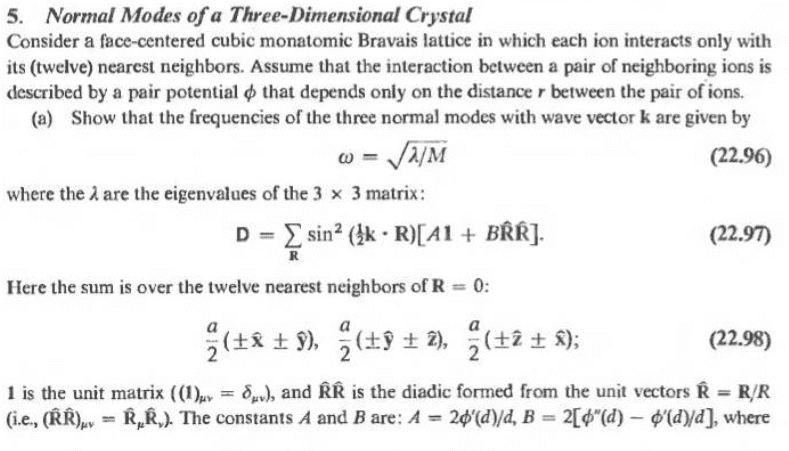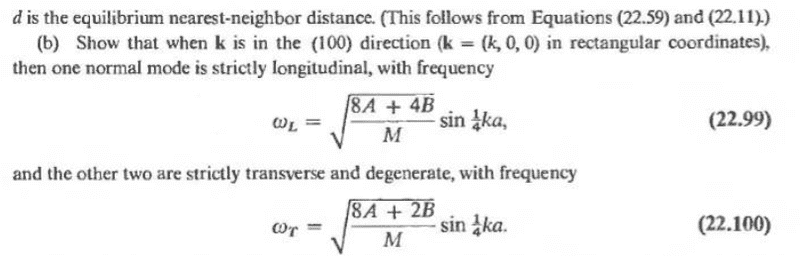# Solid State Physics - Modes of Oscillation of Atom

## Homework Statement[/B]My question is more about the math of the problem. For equation 22.97, how do I construct a 3x3 matrix from a 12 element series? After constructing the matrix should I set the determinant equal to 0 where I will find the determinant has 3 solutions (2 being degenerate)? Also, under 22.98, what does "1 is the unit matrix ((1)uv=diracdelta_uv), and RR is the diadic formed from the unit vectors R=R/R..." mean?
I've never taken linear algebra before so I am unsure how to go from series to matrix, but I know the basics of how to solve them. If it would be easier feel free to send me a link on relevant information regarding matrices.

I don't need much help, but I would appreciate a push in the right direction.